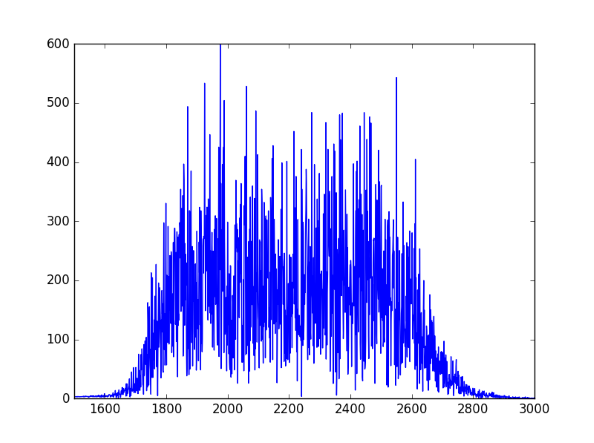# How to create Green noise in Python

This is a follow-on to my previous post on green noise. Here we create green noise with Python by passing white noise through a Butterworth filter.

Green noise is in the middle of the audible spectrum (on the Bark scale), just where our hearing is most sensitive, analogous to the green light, the frequency where our eyes are most sensitive. See previous post for details, including an explanation of where the left and right cutoffs below come from.

Here’s the code:

```from scipy.io.wavfile import write
from scipy.signal import buttord, butter, filtfilt
from scipy.stats import norm
from numpy import int16

def turn_green(signal, samp_rate):
# start and stop of green noise range
left = 1612 # Hz
right = 2919 # Hz

nyquist = (samp_rate/2)
left_pass  = 1.1*left/nyquist
left_stop  = 0.9*left/nyquist
right_pass = 0.9*right/nyquist
right_stop = 1.1*right/nyquist

(N, Wn) = buttord(wp=[left_pass, right_pass],
ws=[left_stop, right_stop],
gpass=2, gstop=30, analog=0)
(b, a) = butter(N, Wn, btype='band', analog=0, output='ba')
return filtfilt(b, a, signal)

def to_integer(signal):
# Take samples in [-1, 1] and scale to 16-bit integers,
# values between -2^15 and 2^15 - 1.
signal /= max(signal)
return int16(signal*(2**15 - 1))

N = 48000 # samples per second

white_noise= norm.rvs(0, 1, 3*N) # three seconds of audio
green = turn_green(white_noise, N)
write("green_noise.wav", N, to_integer(green))

```

And here’s what it sounds like:

Let’s look at the spectrum to see whether it looks right. We’ll use one second of the signal so the x-axis coincides with frequency when we plot the FFT.

```from scipy.fftpack import fft

one_sec = green[0:N]
plt.plot(abs(fft(one_sec)))
plt.xlim((1500, 3000))
plt.show()
```

Here’s the output, concentrated between 1600 and 3000 Hz as expected:## 4 thoughts on “How to create Green noise in Python”

1. A few errors in the code:
1. The value of “right pass” creates a non-positive filter order (and hence an error) — Did you mean instead the following?
right_pass = 0.9*right/nyquist
2. The array “data” does not exist. I believe the last line is rather
write(“green_noise.wav”, N, to_integer(green))

Cheers.

2. dan: Thanks. My working code had gotten out of sync with what I’d written in the blog post.

3. Ari Hartikainen

Instead of using `ba` parameters you could nowdays use “more” accurate `sos` parameters and `sosfiltfilt` function.

4. Eric Fischer

Thanks John. The .wav file you have uploaded sounds like what I get when I apply the upper and lower boundaries you mentioned on my noise generator app. However, according to noise videos on YouTube, green noise sounds like road noise in a car ride and most people find it very pleasing and relaxing as it seems to have a much wider range of frequencies. Of course, the definition seems vague no matter where I have looked. Thanks again!